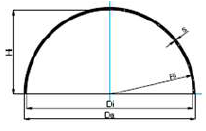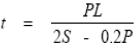Related Resources: pressure-vessel

### Seamless Full-Hemispherical Head ASME Pressure Vessel Equations and Calculator:

ASME Pressure Vessel Design and Engineering

ASME Pressure Vessel Section I: Seamless or Full-Hemispherical Head Equations and Calculator:The thickness of a blank, unstayed, full-hemispherical head with the pressure on the concave side shall be calculated by the following formula:The above formula shall not be used when the required thickness of the head given by the formula exceeds 35.6% of the inside radius. Instead, use the following formula:Where:
t = Minimum thickness of head (in);
P = maximum allowable working pressure (psi);
S = Maximum Allowable Working Stress (psi). According to ASME Section II, Table 1A.

Example:

Calculate the minimum required thickness for a blank, unstayed, full-hemispherical head. The radius to which the head is dished is 7.5 in. The MAWP is 900 psi and the head material is SA 285-C. The average temperature of the header is 570°F.

P = 900 psi;
L = 7.5 in.;
S = 13,800 psi - (SA 285-C at 600°F).

t = [ 900 x 7.5 ] / [ 2 ( 13,800 - 0.2 (900) ]
t = 0.246 in.

Check if this thickness exceeds 35.6% of the inside radius = 7.5 x 0.356 = 2.67 in. It does not exceed 35.6%, therefore the calculated thickness of the head meets the code requirements.